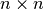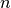• Language: en

# Glossary¶

Akaike information criterion
A method of comparing two similar models by penalising models with a larger number of parameters. See Akaike information criterion on Wikipedia
basin of convergence
A set of initial points that lead to the same local minimum under a given iterative algorithm.
bobyqa optimisation
BOBYQA= Bounded Optimisation by Quadratic Approximation, a none derivative based optimisation method [Powell2009] used by ND method.
C++
C++ is a low level programming language which is automatically used by PoPy for some time critical operations C++ on Wikipedia
categorical covariates
Covariates that indicates membership in one of a set of unordered categories, such as race.
clearance
The volume of the fluid presented to the eliminating organ (extractor) that is effectively completely cleared of drug per unit time. (Definition from [RowlandTozer2012]), also see Clearance on Wikipedia
Compartment Diagram
A graphical visualisation of the compartment model, using nodes for compartments and edges for flows between compartments
confidence intervals
Ranges in which we can be X% confident that a parameter lies.
covariance matrix
A measure of spread for multiple random variables that may be correlated. See Covariance on Wikipedia
covariates
Measured or observed quantities that are read in from the input data file. Signified by a c[X] in the model specification file (which could also be thought of as an abbreviation of “column”). They include information such as ID, time, weight, and also measurements such as drug concentration.
Cython
Cython is an superset of Python that compiles to C++. PoPy uses Cython extensively to process the user config file. See Cython on Wikipedia
DDMoRe
An online repository of PK/PD models see DDMoRe Website
dos prompt
The dos prompt command line in Microsoft Windows. This is the older Windows shell, by default with a black background.
elimination
The removal of a drug from the body.
excretion
The removal of waste substances from the body including unchanged drugs and metabolic products. Most drug products are eliminated through the kidneys.
first order conditional estimation
FOCE is a fitting method in Nonmem that uses a first order approximation of the objective function conditioned on optimised random effects for each individual in the population. For a description of PoPy’s implementation of FOCE see FOCE Fitting Method.
first pass effect
A reduction in the amount of drug entering circulation due to it being metabolised by the liver or gut on its way to the blood system. First pass effect on Wikipedia
fixed effects
A population-level parameters (usually means) that describe an average from which individuals deviate in a random way, though where the nature of the randomness is known. Signified by f[X] in the model specifications file.
Graphviz
Graphviz is open source software used to create Compartment Diagram in PoPy. See Graphviz on Wikipedia
hessian
Thematrix of second derivatives of a function ofvariables. Contains information that describes the shape of the surface at a given point (the minimum, for example).
HTML
Hyper Text Markup Language used on the web and by PoPy to generate summary output. See HTML on Wikipedia
importance sampling
A method of sampling from a complex distribution by first sampling from a simpler distribution and re-weighting with the ratio of the complex and simpler Wikipedia: <Probability_density_function>. See Importance Sampling on Wikipedia.
initial value problem
The ordinary differential equations typically solve a dynamic system which has a defined input state and then the system evolves over time according to the ordinary differential equation system. This type of integration problem, typical in PK/PD, is known as a Initial Value Problem.
iterative two stage
ITS is a fitting method in Nonmem that optimises the objective function by switching between optimising the fixed effects and random effects.
joint optimisation and estimation
JOE is PoPy’s original fitting method see JOE Fitting Method, it optimises the same objective function as FOCE and ITS and is most similar to ITS in terms of fitting performance.
Laplace approximation
A method of approximating integrals. See Laplace method on Wikipedia. This objective function is used by LAPLACE and an approximation is used by JOE, FOCE and ITS fitting methods.
laplace fitting method
A fitting method that uses the Laplace approximation as an objective function. Note JOE, FOCE and ITS use a related, but less computationally expensive objective function.
Likelihood
The conditional probability, p(D|M), of observing data D given a hypothesized model M. This expresses the plausibility of model M given data D, but is a probability distribution over D rather than M. As a result, it cannot be used to compare different models, only different parameter values for the same model. Likelihood on Wikipedia
LSODA
Numerical ordinary differential equation solver [Radhakrishnan1994] available in PoPy, see Example ODE_SOLVER using CPPLSODA.
mass balance
The principal that matter cannot be created or destroyed within a compartment model, apart from deliberate inputs (e.g doses) and sink compartments that model excretion from the body. See Mass Balance on Wikipedia.
metabolism
Process by which drug is chemically transformed into another substance. Takes place primarily in the liver.
Microsoft Windows
A popular operating system for personal computers.
mixed effect model
A structural model that uses both fixed effects and random effects to model population parameters. In practise, all models contain at least one fixed effect, so the key feature is the use of random effects to allow parameters to vary between subjects in the population.
model parameters
Person-specific PK/PD parameters, usually defined as a function of the fixed effects, random effects and measured covariates. Signified by m[X] in the model specification file.
Monolix
Matlab based PK/PD modelling software. See http://lixoft.com/products/monolix/
MPI
Message Passing Interface. A protocol for parallelising software by passing information between processors. See Message Passing Interface on Wikipedia
noise
Random displacements added to a signal. See Signal Processing Noise on Wikipedia
none derivative estimation
ND is PoPy’s newest fitting method see ND Fitting Method, it optimises the same objective function as FOCE and uses the derivative based FOCE fitting method, but also utilises the none derivative BOBYQA algorithm.
Nonmem
Nonmem (NONlinear Mixed Effect Modelling) is a Fortran based system for PK/PD modelling. [Bauer2009]
objective function
The fixed effects and random effects of a model are estimated by minimising the objective function, which is equivalent to maximising the likelihood of the model given the observations.
observations
The observed values to be modelled, also known as the dependent variable. These measurements (either synthetic or real) are signified by c[X] in the PREDICTIONS section of a PoPy script file.
ordinal covariates
Covariates derived from a discretisation of a continuum such that values have a definite order, such as the East Coast Oncology Group status that ranges from 0 (normal) to 4 (most severe).
ordinary differential equations
Multiple differential equations, each with one independent variable. See Ordinary differential equation on Wikipedia
powershell prompt
The powershell prompt command line in Microsoft Windows. This is the newer Windows shell, by default with a blue background.
practically identifiable
A parameter of a model is practically identifiable or estimable, if the true value can be estimated from a finite amount of data. See Identifiability Analysis on Wikipedia
practically unidentifiable
A parameter that is not practically identifiable
predictions
The value the model calculates for a given observations, usually a conversion to concentrations via division by the volumes of the compartments. Signified by p[X] in the model specification file.
product key
The PoPy product key is the unique key that identifies the the current licence. It has a form like ‘XXXX-XXXX-XXXX-XXXX-XXXX-XXXX-XXXX’. See PoPy Activation.
Python
Python is a general purpose programming language used in PoPy scripts and to implement PoPy itself. See Python on Wikipedia
R
R is open source statistical software used extensively in the PK/PD community. See R on Wikipedia
random effects
Deviation from the population-level fixed parameters, with defined distribution parameters. Signified by r[X] in the model specification file.
shrinkage
The tendency to for random effects to shrink towards the mean value when data are sparse.
solutions
Solutions are defined by a .pyml file containing links to .csv files that determine a set of f[X], r[X], m[X], s[X], p[X] variables that represent a candidate solution to a PK/PD model fitting problem.
Sphinx
Documentation system used by PoPy and many other Python projects to generate .html and .pdf files. See Sphinx on Wikipedia
state parameters
The amount - not concentration - of drug in each compartment of the compartment model. Signified by s[X] in the model specification file.
stochastic approximation expectation maximisation
SAEM is a probabilistic fitting method originally implemented in Monolix and also available in Nonmem.
structurally identifiable
A parameter of a model is structurally identifiable, if given an infinite amount of data the true underlying parameter value is recoverable. See Identifiability Analysis on Wikipedia
structurally unidentifiable
A parameter that is not structurally identifiable
symmetric positive definite
A symmetric positive definite matrix is a matrix whose eigenvalues are all positive. It is the matrix equivalent of having a real valued square root. In PK/PD models a population covariance matrix is required to be symmetric positive definite. See Matrix Definiteness on Wikipedia
variance
A measure of spread for a random variable. See Variance on Wikipedia
visual predictive check
Given a set of f[X] values and a model, new p[X] values are simulated which can then be compared with original c[X] data on a graph.
volume of distribution
The volume (or volume of distribution) is the theoretical volume that a compartment would need to have to give the concentration of drug found in the blood plasma. See Volume of Distribution on Wikipedia
YAML
A simple markup language used by PoPy Script File Formats. See YAML on Wikipedia